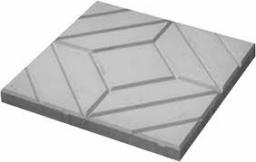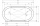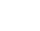# Rhombus 2

Calculate the area of rhombus which has a height v=48 mm and shorter diagonal u = 60 mm long.

Correct result:

S =  2400 mm2

#### Solution:

$a = x+y \ \\ 60^2 = 48^2 + y^2 \ \\ y= \sqrt{ 60^2 - 48^2} = 36 \ mm \ \\ \ \\ a^2 = x^2+48^2 \ \\ (x+y)^2 = x^2+48^2 \ \\ x^2+2xy +y^2 = x^2+48^2 \ \\ 2xy+y^2 = 48^2 \ \\ 2\cdot 36 \cdot x = 48^2 - 36^2 \ \\ x = 14 \ mm \ \\ \ \\ a = x+y = 14 + 36 = 50 \ mm \ \\ \ \\ S = a v = 50 \cdot 48 = 2400 \ \text{mm}^2 \ \\$We would be very happy if you find an error in the example, spelling mistakes, or inaccuracies, and please send it to us. We thank you!Tips to related online calculators
Pythagorean theorem is the base for the right triangle calculator.

#### You need to know the following knowledge to solve this word math problem:

We encourage you to watch this tutorial video on this math problem:

## Next similar math problems:

• 9-gon pyramidCalculate the volume and the surface of a nine-sided pyramid, the base of which can be inscribed with a circle with radius ρ = 7.2 cm and whose side edge s = 10.9 cm.
• Surface of the coneCalculate the surface of the cone if its height is 8 cm and the volume is 301.44 cm3.
• CalculateCalculate the surface and volume of the cone that results from the rotation of the right triangle ABC with the squares 6 cm and 9 cm long around the shorter squeegee.
• Volume of the coneCalculate the volume of the cone if the content of its base is 78.5 cm2 and the content of the shell is 219.8 cm2.
• RunningThe length of the inner edge of the running oval is 400 m. The straight sections measure 90 m. Calculate the dimensions of the oval - the rectangle where this oval can fit.
• Isosceles triangleIn an isosceles triangle ABC with base AB; A [3,4]; B [1,6] and the vertex C lies on the line 5x - 6y - 16 = 0. Calculate the coordinates of vertex C.The height of a regular quadrilateral prism is v = 10 cm, the deviation of the body diagonal from the base is 60°. Determine the length of the base edges, the surface, and the volume of the prism.
• How manyHow many m2 of copper sheet is needed to replace the roof of a conical tower with a diameter of 13 meters and a height of 24 meters, if we count 8% of the material for bending and waste?
• Edge cFind the edge c of cuboid if an edge a = 20 mm, b = 30 mm and surface area S = 8000 mm2.
• Sphere parts, segmentA sphere with a diameter of 20.6 cm, the cut is a circle with a diameter of 16.2 cm. .What are the volume of the segment and the surface of the segment?
• Area and perimeter of rectangleThe content area of the rectangle is 3000 cm2, one dimension is 10 cm larger than the other. Determine the perimeter of the rectangle.
• The volumeThe volume of the sphere is 1 m ^ 3, what is its surface?
• Isosceles trapezoidFind the area of an isosceles trapezoid, if the bases are 12 cm and 20 cm, the length of the arm is 16 cm
• Tangents to ellipseFind the magnitude of the angle at which the ellipse x2 + 5 y2 = 5 is visible from the point P[5, 1] .
• Find the 15Find the tangent line of the ellipse 9 x2 + 16 y2 = 144 that has the slope k = -1
• Digging a pitThe pit has the shape of a regular quadrilateral truncated pyramid. The edges of the bases are 14m and 10m long. The sidewalls form an angle of 135° with a smaller base. Determine how many m3 of soil were excavated when digging the pit?
• Thousand ballsWe have to create a thousand balls from a sphere with a diameter of 1 m. What will be their radius?